Question

Using the tables for water, determine the specified property data at the indicated states.

(a) At p = 3 bar, v = 0.35 m3/kg, find T in °C and u in kJ/kg.
(b) At T = 320°C, v = 0.33 m3/kg, find p in MPa and u in kJ/kg.
(c) At p = 28 MPa, T = 400°C, find v in m3/kg and h in kJ/kg.
(d) At T = 10°C, v = 70 m3/kg, find p in kPa and h in kJ/kg.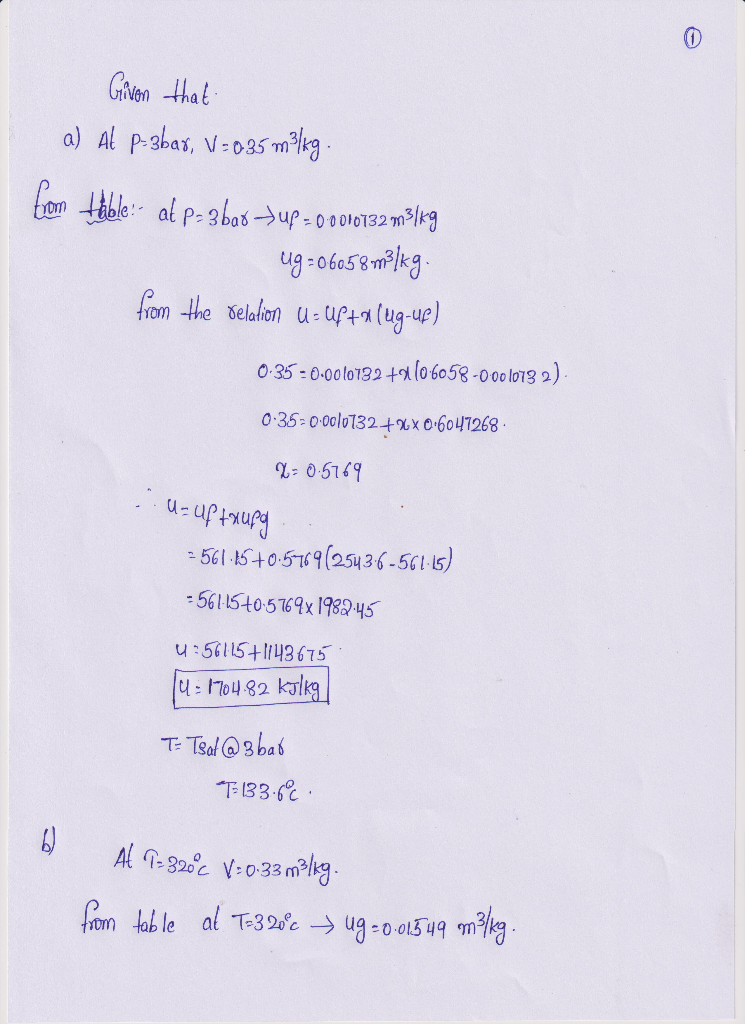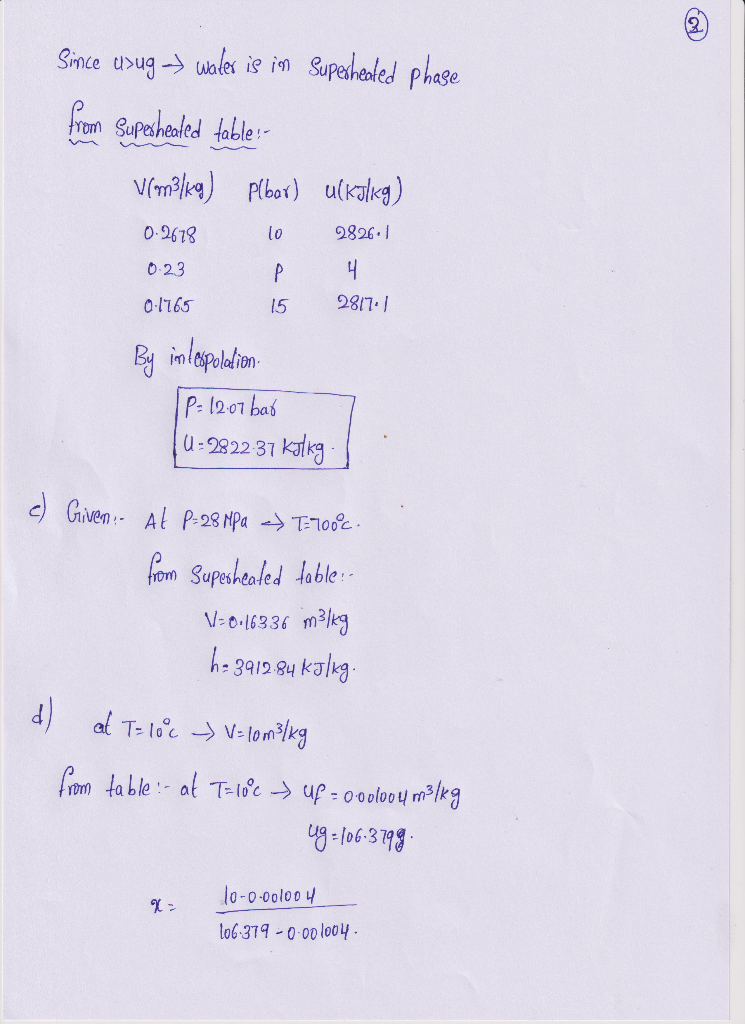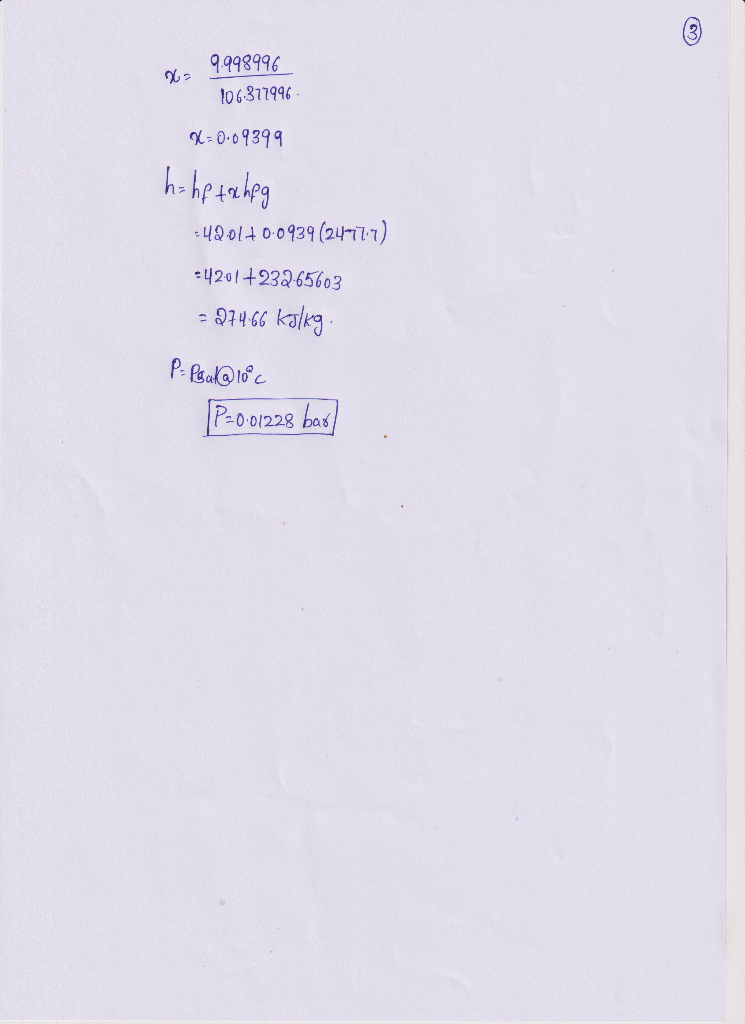#### Earn Coins

Coins can be redeemed for fabulous gifts.

Similar Homework Help Questions
• ### Q:1 Using the tables for water determine the specified property data at the indicated state. For...

Q:1 Using the tables for water determine the specified property data at the indicated state. For H2O at p = 0.7 MPa and v = 0.57 m3/kg, find h in kJ/kg. Note: Round up the answer to 2 decimal places. Q:2 Using the tables for water determine the specified property data at the indicated state. For H2O at p = 0.7 MPa and v = 0.57 m3/kg, find u in kJ/kg. Note: Round up the answer to 2 decimal places....

• ### Problem 3.10 (water properties). For H20, determine the specified property at the indicated state. Locate the...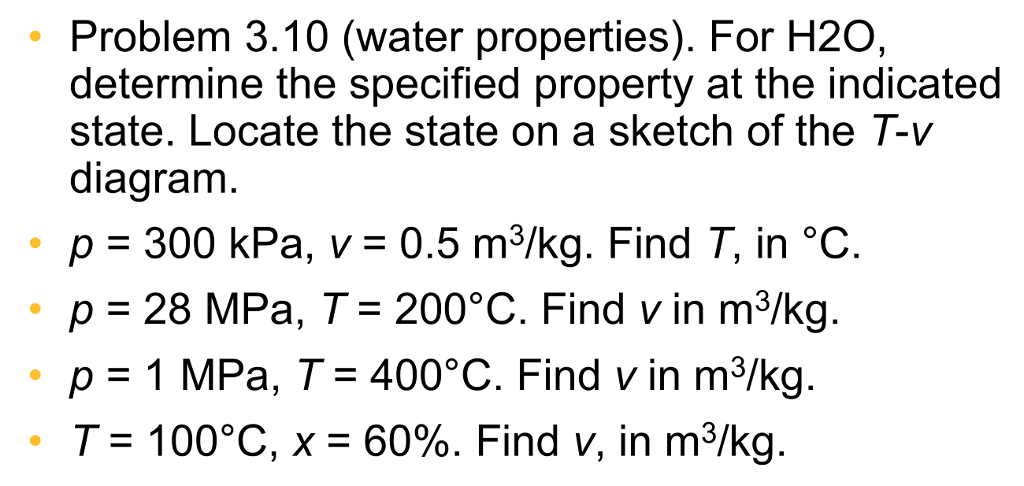Problem 3.10 (water properties). For H20, determine the specified property at the indicated state. Locate the state on a sketch of the「-v diagram , p-300 kPa, v:0.5 m3/kg. Find T, in °C. p 28 MPa, T- 200°C. Find v in m3/kg p 1 MPa, T 400°C. Find v in m3/kg. 、 7-100"C, x-60%. Find v, in m3/kg.

• ### Question 1 Using the tables for water determine the specified property data at the indicated state....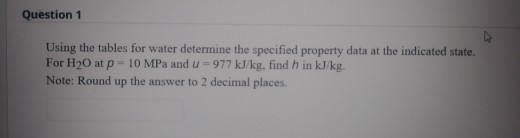Question 1 Using the tables for water determine the specified property data at the indicated state. For H20 at p = 10 MPa and u- 977 kJ/kg, find h in kJ/kg. Note: Round up the answer to 2 decimal places.

• ### Thermodynamics help

Using the tables for water, determine the specified property data at the indicated states. In each case, locate the state by hand on sketches of the p–y and T–ydiagrams. (a) At p 5 3 bar, y 5 0.5 m3/kg, find T in °C and u in kJ/kg. (b) At T 5 320°C, y 5 0.03 m3/kg, find p in MPa and u in kJ/kg. (c) At p 5 28 MPa, T 5 520°C,find y in m3/kg and h in kJ/kg....

• ### Using the tables for water determine the specified property data at the indicated state. For H2O...

Using the tables for water determine the specified property data at the indicated state. For H2O at T = 6 °C and X = 33 %, find u in kJ/kg. Note: Round up the answer to 2 decimal places.

• ### 3.6 WP For H20, determine the specified property at the indicated state. Locate the state on a sk...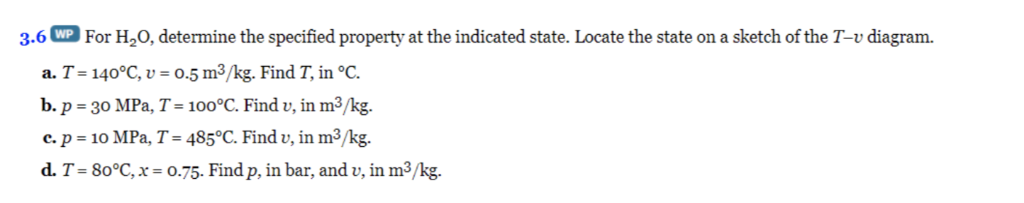3.6 WP For H20, determine the specified property at the indicated state. Locate the state on a sketch of the T-v diagram. a. T- 140°C, .5m3/kg. Find T, in °C b. p-30 MPa, 7-100°C Find v, in m3/kg. c. p 10 MPa, T- 485°C. Find v, in m3/kg. d. T-80°C, x o.75. Find p, in bar, and v, in m3/kg. 3.6 WP For H20, determine the specified property at the indicated state. Locate the state on a sketch of the...

• ### Problem 3.006 SI For H20, determine the specified property at the indicated state. (a) T =...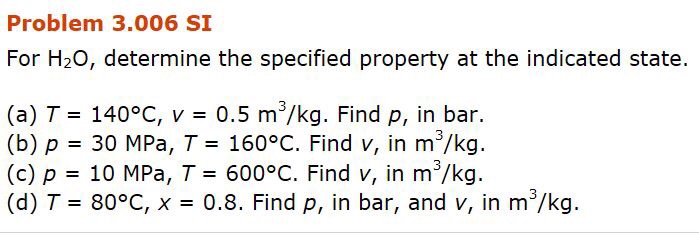Problem 3.006 SI For H20, determine the specified property at the indicated state. (a) T = 140°C, v = 0.5 m3/kg. Find p, in bar. (b) p = 30 MPa, T= 160°C. Find v, in m3/kg. (c) p = 10 MPa, T= 600°C. Find v, in ms/kg. (d) T= 80°C, x = 0.8. Find p, in bar, and v, in m3/kg.

• ### 1. Using the tables for water, determine the specific entropy at the indicated states, in kJ/kg.K...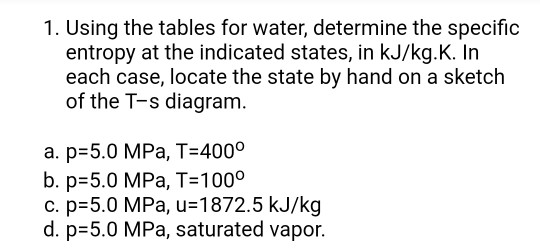1. Using the tables for water, determine the specific entropy at the indicated states, in kJ/kg.K. In each case, locate the state by hand on a sketch of the T-s diagram a. p-5.0 MPa, T-4000 b. p 5.0 MPa, T 100o c. p 5.0 MPa, u-1872.5 kJ/kg d. p 5.0 MPa, saturated vapor. 1. Using the tables for water, determine the specific entropy at the indicated states, in kJ/kg.K. In each case, locate the state by hand on a sketch...

• ### EASY Thermodynamics - using tables to evaluate properties

Using the tables for water determine the specified property data at the indicated states. In each case, locate the state on sketches of the p-v and T-vdiagrams.a) p=2 MPa, T=300 degrees C. Find u, in kJ/kg.b) p=2.5 MPa, T=200 degrees C. Find u, in kJ/Kg.c) T=170 degrees F, x=50%. Find u in Btu/lb.d) p=100 lbf/in^2, T=300 degrees F. Find h in Btu/lbe) at p=1.5 MPa, v=0.2095 m^3/kg. Find h, in kJ/kg.

• ### Click Submit to complete this assessment. Question 2 Using the tables for water determine the specified...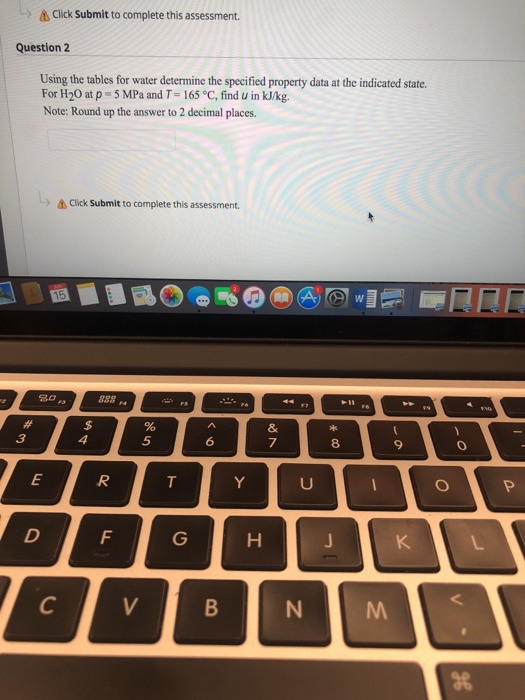Click Submit to complete this assessment. Question 2 Using the tables for water determine the specified property data at the indicated state. For H20 at p 5 MPa and T 165 °C, find U in kJ/kg. Note: Round up the answer to 2 decimal places. Click Submit to complete this assessment. 5